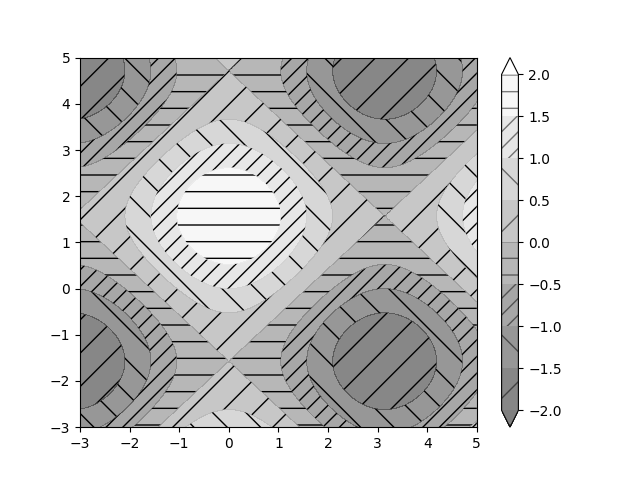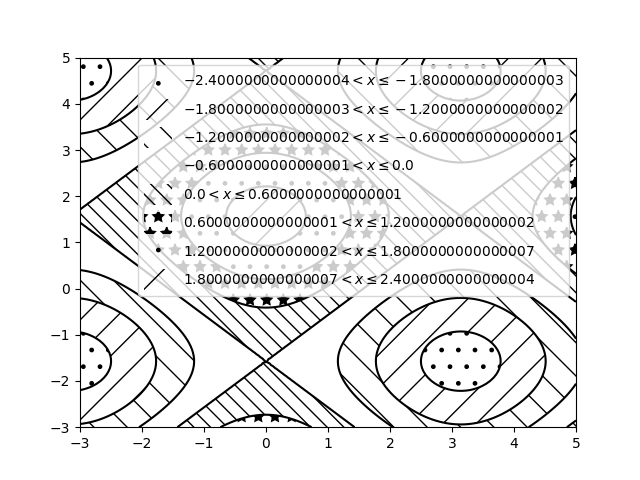Travis-CI:# Contourf Hatching¶

Demo filled contour plots with hatched patterns.

```import matplotlib.pyplot as plt
import numpy as np

# invent some numbers, turning the x and y arrays into simple
# 2d arrays, which make combining them together easier.
x = np.linspace(-3, 5, 150).reshape(1, -1)
y = np.linspace(-3, 5, 120).reshape(-1, 1)
z = np.cos(x) + np.sin(y)

# we no longer need x and y to be 2 dimensional, so flatten them.
x, y = x.flatten(), y.flatten()
```

Plot 1: the simplest hatched plot with a colorbar

```fig = plt.figure()
cs = plt.contourf(x, y, z, hatches=['-', '/', '\\', '//'],
cmap=plt.get_cmap('gray'),
extend='both', alpha=0.5
)
plt.colorbar()
```Plot 2: a plot of hatches without color with a legend

```plt.figure()
n_levels = 6
plt.contour(x, y, z, n_levels, colors='black', linestyles='-')
cs = plt.contourf(x, y, z, n_levels, colors='none',
hatches=['.', '/', '\\', None, '\\\\', '*'],
extend='lower'
)

# create a legend for the contour set
artists, labels = cs.legend_elements()
plt.legend(artists, labels, handleheight=2)

plt.show()
```Total running time of the script: ( 0 minutes 0.082 seconds)

Gallery generated by Sphinx-Gallery